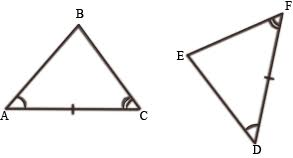### Congruence of Triangles

Two triangles are congruent if and only if one of them can be made to superpose on the other, so as to cover it exactly. let ∆ABC and DEF be two congruent triangles. Then, we can superpose ∆ABC on  ∆DEF, in some order.

### Congruence relation

From the definition of congruence of two triangles, we obtain the following results:
• Every triangle is congruent to itself i.e., ∆ABC ≅ ABC.
• If  ABC ≅  ∆DEF, then ∆DEF = ∆ABC.
• If  DEF ≅  ∆ABC, then ∆ABC = ∆DEF.

### Sufficient Conditions (Criteria) For Congruence of Triangles

Two triangles are congruent if and only if there exists a correspondence between there vertices such that the corresponding sides and corresponding angles of two triangles are equal i.e. six equalities hold good, three of the corresponding sides, and three of the corresponding angles. If three properly chosen conditions are satisfied, then the other three are automatically satisfied.

### Side-angle-side (SAS)

Two triangles are said to be congruent if two sides and the included angle of one are equal to the corresponding sides and the included angle of the other triangle.
Angle opposite to two equal sides of triangles are equal, in the figure below ∠C = ∠Q and ∠B = ∠S.
Note: Equal angle should be the angle included between the equal sides.

### Angle-Side-Angle (ASA)

Two triangles are congruent if two angles and the included side of one triangle are equal to the corresponding two angles and the included side of the other triangle.### Angle-Side-Angle (AAS)

If any two angles and a non-included side of one triangle are equal to the corresponding angles and side of another triangle, then the two triangles are congruent.### Side-Side-Side (SSS)

Two triangles are congruent if three sides of one triangle are equal to the corresponding three sides of the other triangle.

### Right Angle-Hypotenuse-Side (RHS)

Two right triangles are congruent if the hypotenuse and one side of one triangle are respectively equal to the hypotenuse and one side of the other triangle.
CPCTC

#### ∠A ≅ ∠P, ∠B ≅ ∠Q, ∠C ≅ ∠R, AB ≅ PQ, BC ≅ QR, AC ≅ PR.

We probably knew many of these before CPCTC because that's how we proved triangles were congruent in the first place.
Often we use CPCTC as the last step of our proof to conclude we were trying to prove.

Some Example Problems
Example 1: Let ABCD be a parallelogram and AC be one of its diagonals. What can you say about triangles ABC and CDA? Explain your answer.

Solution:
• In a parallelogram opposite angle are equal so we have Angle(A) = Angle(B).
• It is given that AB = CD and BC = DA so by SAS congruent property ∆ABC ≅ ∆ CDC.